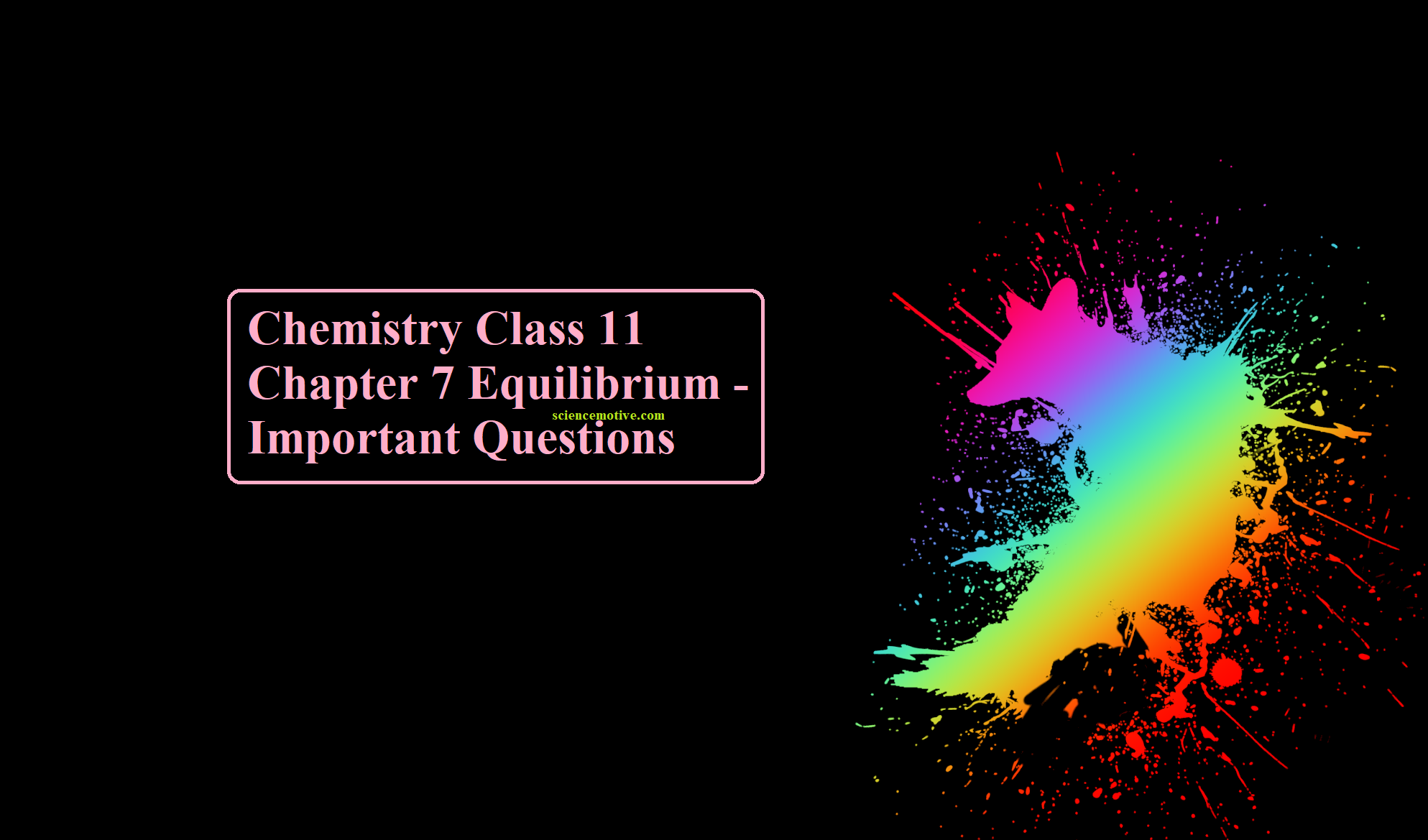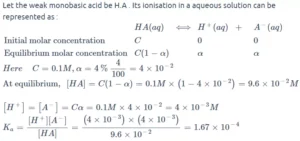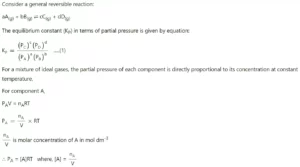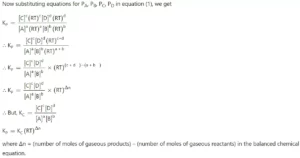# Chemistry Class 11 Chapter 7 Equilibrium – Important Questions# Chemistry Class 11 Chapter 7 Equilibrium – Important Questions

Chemistry Class 11 Chapter 7 Equilibrium

Que 1. What is the effect of catalyst on equilibrium constant Kc?
Ans 1. A catalyst does not affect the equilibrium constant because it speeds up both forward and backward reactions to the same extent.

Que 2. What will be the pH of the Na2SO4 solution?
Ans 2. Na2SO4 is salt of a strong base, thus its aqueous solutions will be neutral. Therefore, its pH will be 7.

Que 3. What happens to the equilibrium
PCl5 (g)
⇌ PCl3 (g) + Cl2 (g) if nitrogen is added to it
(i) at constant volume
(ii) at constant pressure?
Ans 3
. (i) The state of equilibrium remains unaffected.

(ii) Dissociation increases, i.e., the equilibrium shifts forward.

Que 4. What happens to the ionic product of water if some acid is added to it?
Ans 4. Ionic products will remain unchanged.

Que 5. If Qc > Kc, what would be the type of reaction?
Ans 5. If Qc > Kc, the reaction will proceed in the direction of the reactants (reverse reactions).

Que 6. What is meant by the statement ‘Equilibrium is dynamic in nature?
Ans 6. That stage of the reaction at which reaction will not stop rather than stop it will be in a continuous manner then the equilibrium is said to be dynamic in nature. In this case, the reaction appears to stop because the rate of forwarding reaction is equal to the rate of backward reaction.

Que 7. Write the relationship between Kp and Kc for the gaseous reaction – N2 + O2 ⇌ 2NO.
Ans 7. Kp = Kc as ∆n is zero for the above-said reaction.

Que 8. Classify the following species as Lewis acids and Lewis bases
NH3, BF3, SnCl4, C5H5N, CO, and Ni2+
Ans 8. Lewis acids: BF3, SnCl4, Ni2+
Lewis basis: NH3, C5H5N, CO

Que 9. Ionic product of water at 310 K is 2.7 × 10–14. What is the pH of neutral water at this temperature?
Ans 9. Kw = [H3O+] [OH] = 2.7 × 10–14
[H3O+] = [OH]
[H3O+]2 = 2.7 × 10–14 = Kw
[H3O+] = 1.643 × 10–7
pH = – log[H3O+] = – log(1.643 × 10–7)
= – 0.2156 – 7 = 6.75

Que 10. Write the relation between Kc and Kp for the reaction
PCl5 (g)
⇌ PCl3 (g) + Cl2 (g)
Ans 10.
Δn = 2 – 1 = 1

Hence Kp = Kc × (RT)Δn = K × RT.

Que 11. Can a catalyst change the position of equilibrium in a reaction?
Ans 11. No, a catalyst cannot change the position of equilibrium in a chemical reaction. A catalyst, however, affects the rate of reaction.

Que 12. The equilibrium constant for the reaction H2O + CO H2 + CO2. Is 0.44 at 1260 K. What will be the value of the equilibrium constant for the reaction?
2H2(g) + 2CO(g) 2CO(g) at 1260 K.
Ans 12. The value of equilibrium gets reversed and also becomes double which can be represented as
Kc = (1/0.44)2 = 5.16

Que 13. The concentration of hydrogen ion in a sample of soft drink is 3.8 × 10–3M. What is its pH?
Ans13. pH = – log[3.8 × 10–3] = – {log[3.8] + log[10–3]}
= – {(0.58) + (– 3.0)} = – { – 2.42} = 2.42
Therefore, the pH of the soft drink is 2.42 and it is acidic

Que 14. A weak monobasic acid is found to be 4 % ionized at 0.1 M concentration. Since no equilibrium is present in the strong electrolytes.
Ans 14.Que 15. What is the pH of our blood? Why does it not change in spite of the variety of foods and Spices we eat?
Ans 15.
The pH of our blood is about 7.4. It remains constant because blood is a buffer.

Que 16. Justify the statement that water behaves like acid and also like a base on the basis of the protonic concept
Ans 16. Water ionizes as H2O + H2O H3O+ OH
With strong acid, water behaves as a base and accept the proton given by the acid e.g.
HCl + H2O Cl + H3O+
While with a strong base, water behaves as an acid by liberating a proton e.g.
H2O + NH3 NH4+ + OH.

Que 17. Define Buffer solution.
Ans 17. The solutions which resist change in pH on dilution or with the addition of small amounts of acid or alkali are called Buffer solutions.

Que 18. Write the equilibrium constant for the following equation
aA + bB cC + dD

Ans 18. The equilibrium constant of this reaction can be written as
Kc = [C]C[D]d
[A]a[B]b
Here A, B, C, and D are said to be the equilibrium concentrations of the reactants and products.

Que 19. Give the relation Kp = Kc (RT)Δn
Ans 19.Que 20. What happens when temperature increases for a reaction?
Ans 20: The value of the equilibrium constant will decrease with the rise in temperature.

Chemistry Class 11 Chapter 7 Equilibrium